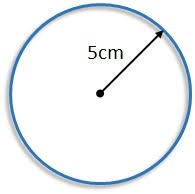# C Program to find the Area Of a Circle

C Program to find the Area of a Circle – In this particular article, we will detail in how to find the area of a circle in C Programming. There are multiple ways explained in this piece on how to find the area of a circle.

Check out the suitable examples and the sample programs mentioned to explain the c programming code to calculate the area of a circle. The compiler has been added to help you with the solution for the question of concern.

The ways in which the area of a circle is calculated in this article are as follows:

• Using Standard Method
• Using Functions
• Using Pointers
• Using Macros

As we all know, circles are the combination of infinite points forming a locus which is equidistant from a single point. The locus is known as the circumference.

The point which is inside the circle that is equidistant to the circumference is known as the centre of the circle. The distance between the centre of the circle and the circumference is known as the radius of the circle.

A circle looks like this:As we can see in the image uploaded above, the radius of the circle is 5cm.

Thus, the area of the circle is

Area = 2 * pi * (sq)r = 2 * pi * (sq)5

Area = 50pi = 157.08 sq.cm

Thus, the ways to calculate the area of a circle mentioned are as follows:

## Using Standard Method

• To calculate the area of a circle, we need the radius.
• The value of radius will store into the variable “r”.
• By substituting the “r” value in the formula, we will get the area.
Output:

### Using Functions

• We are calling the function as area(r) which is having int type of argument.
• The called function float area(int r) will calculate the area and return the value, that will return value assigned to the variable “a”.
Output:

#### Using Pointers

• We are calling the function by passing references as arguments using area(&r,&a).
• The called function area(int *r, float *a) will retrieve the values at that given addresses and calculate the area and that area value will be stored into the pointer variable “a”.
Output:
##### Using Macros
• area(r) is the symbolic name to the expression (22*r*r)/7
• area(r)replaced with that expression given at #define.
Output:
x

## C Program To Print Number Of Days In A Month | Java Tutoring

C program to input the month number and print the number of days in that ...